# Thermo-Mechanical Modeling and Simulation of Impact and Solidification of an Aluminum Particle

Thermo-Mechanical Modeling and Simulation of Impact and Solidification of an Aluminum Particle
Laboratory of Mechanics, Physics, and Mathematical Modeling (LMP2M), University of Medea, Medea 26000, Algeria

Department of Mechanical Engineering, Faculty of Technology, University of Medea, Medea 26000, Algeria

Department of Mechanical and Mechatronics Engineering, Afe Babalola University, Ado Ekiti 360101, Nigeria

Electrical Engineering Technical College, Middle Technical University, Baghdad 10001, Iraq

Control and Systems Engineering Department, University of Technology, Baghdad 10066, Iraq

Department of Industrial Engineering, University of Parma, Parco Area delle Scienze 181/A, Parma 43124, Italy

Department of Technology, University Center Salhi Ahmed Naama (Ctr. Univ. Naama), P.O. Box 66, Naama 45000, Algeria

College of Engineering, National University of Science and Technology, Dhi Qar, Iraq

Corresponding Author Email:
menni.younes@cuniv-naama.dz
Page:
389-397
|
DOI:
https://doi.org/10.18280/mmep.100201
19 January 2023
|
Revised:
7 March 2023
|
Accepted:
13 March 2023
|
Available online:
28 April 2023
| Citation

OPEN ACCESS

Abstract:

In the thermal spray procedure described in this research, a single aluminum particle is deposited and flattened using thermomechanical modeling and simulation. The explicit software Abaqus is used to conduct the numerical analysis. In this approach, the thermomechanical characteristics of the particle and the substrate are regarded as temperature-dependent. Only heat transmission through conduction is taken into account in this investigation, and a variable thermal contact conductance is employed. To start, we compare the current model to the experimental as well as numerical data that are mentioned in the literature. During the particle impact, the evolution of temperature, displacement, Von-Mises stress, and equivalent plastic strain as functions of time are assessed. In addition, the present model that considers the thermal and mechanical interactions between the particle and the substrate has been found to assist in comprehending the mechanism of lamella formation and heat transfer during thermal spraying.

Keywords:

numerical simulation, thermomechanical analysis, conduction heat transfer, central-difference integration, internal flux vector

1. Introduction

Thermal spray coating is a widely used method in various industries such as aerospace, automotive, and thermal barrier sectors. The process involves the fine spraying of metals, alloys, ceramics, polymers, and composites into a plasma or oxy-fuel flame, melting them until they reach their melting point, and then impacting and bonding them to a pre-treated substrate. Porosity, oxide content, macro- and micro-hardness, binding strength, and surface roughness are a few of the variables that affect coating quality . Molding, welding, thermal spraying, among other industries, use the solidification with phase change process. Because lamella formation occurs quickly and at a micrometer scale in thermal spraying, the deformation and solidification of the deposited particles is still a complex phenomenon. Numerous numerical studies and experiments conducted recently or even earlier have concentrated on the solidification process in thermal spraying. The droplet and substrate experience the majority of the heat transfer during impact and flattening. By resolving a one-dimensional Stefan problem, Madjeski  has examined the droplet solidification analytically. The effects of multiple ceramic droplets with liquid/solid phase change have been numerically explored by Le Bot et al. . It was discovered that the temperature of the substrate exhibits a delay before rising when the first particle impacts it. The findings also demonstrated the significance of the initial contact between droplets and substrate due to higher thermal contact resistance. In their research, Shukla et al.  devised a 2D axisymmetric model that employed the volume of fluid method to analyze the behavior of a molten pure metal droplet upon impact, spreading, and rapid solidification on a substrate. The results indicate that the liquid component of the droplet extends without solidifying when the temperature at the droplet-substrate interface exceeds the nucleation temperature. As the temperature between the droplet and the substrate reaches the nucleation temperature, a thin solid layer begins to form on the substrate.

It is well knowledge that alloys solidify very differently from pure metals. A variety of temperatures cause an alloy to solidify, resulting in the development of the mushy area (solid-liquid region). To study the solidification of cast iron droplets and the melting and reconsolidation of aluminum substrate during spraying, Xing et al.  created a 1D heat transport model. The observation revealed that a higher initial temperature of the substrate promotes deeper melting of its surface, resulting in a robust metallurgical bond between the cast iron splat and the aluminum substrate.

Experimental research on the effects of molten solder droplets with an average diameter of 78 μm was conducted by Haferl and Poulikakos . According to the findings, the shape of the 1st droplet that has already solidified significantly affects the collision and solidification of the 2nd droplet. Additionally, the temperature of the substrate affects the 2nd droplet's overall solidification time in a non-monotonic manner. According to Zhao's experimental findings , particles projected onto aluminum harden more quickly than those projected onto stainless steel. The coatings become porous as a result of the quick solidification.

In the thermal spraying process, air is trapped in crevices at the droplet's contact surface with the substrate when it comes into contact with the substrate, causing a discontinuity in the temperature distribution . The thermal contact resistance (TCR), which has been extensively researched and whose value relies on surface quality, contact pressure, and material parameters, is used to describe this discontinuity. Heichal and Chandra  developed an analytical model and conducted experiments to predict the thermal contact resistance of molten metal droplets on a solid surface. The research showed that an increase in surface roughness led to a rise in thermal contact resistance, whereas an increase in impact velocity resulted in a decrease in thermal contact resistance.

Chung and Rangel  and Zhang et al.  used mathematics to investigate the effects of thermal contact resistance on the deposition and solidification of metal droplets. The splat size and solidification time were found to be significantly influenced by the contact resistance. The splat creation during thermal spraying has been mathematically explored by Zhang et al. . If the interfacial heat transfer coefficient is low, the findings suggest that the nucleation delay time may exceed the spreading time.

There are two types of droplet impact, spreading, and solidification numerical models. In the 1st way, the droplet is considered as a fluid, and the droplet-air interaction is monitored using the volume of fluid method (VOF) [13-20]. The 2nd way is as follows: the droplet is viewed as a solid with temperature-dependent thermomechanical characteristics . Fardan and Ahmed  utilized the abaqus/explicit program to examine the development of residual stress in a thermally sprayed yttrium-stabilized zirconia coating on a stainless-steel substrate. According to the outcomes, the residual stresses in the substrate are compressive, whereas those in the coating are predominantly tensile if the substrate is not yet cooled.

The current study's goal is to numerically explore how an aluminum particle interacts with and deposits itself on a tool steel substrate during thermal spraying. The study focuses on the testing of a method that simulates the impact and distribution of an aluminum particle using a thermomechanical model. The thermo-mechanical model takes into account material characteristics that vary with temperature and uses a correlation of changeable thermal contact conductance. The abaqus/explicit software is used to solve the set of governing equations.

2. Mathematical Modeling

The heat transfer equations are integrated using the explicit forward-difference time integration rule in ABAQUS/Explicit as follows :

$\theta_{(i+1)}^N=\theta_{(i)}^N+\Delta t_{i+1} \dot{\theta}_{(i)}^N$     (1)

where, $\theta^N$ denotes the temperature at node N and i denotes the increment in a certain dynamic step. At the start of the increment, the values of $\dot{\theta}_{(i)}^N$ are determined by:

$\dot{\theta}_{(i)}^N=\left(C^{N J}\right)^{-1}\left(P_{(i)}^J-F_i^J\right)$     (2)

where, PJ denotes the applied nodal source vector, FJ is the internal flux vector, and CNJ denotes the lumped capacitance matrix.

$[c]=\int_V[N]^T \rho\left(\frac{d H}{d T}\right)^t[N] d V$     (3)

According to the research , the specific heat cp in the zone of solidification is as follows:

$c_p(T)=\frac{d H}{d T}-\frac{H_f}{\left(T_{l i q}-T_{s o l}\right)}$     (4)

The liquidus temperature, solidus temperature, and latent heat of fusion are represented by the letters Tliq, Tsol, and Hf, respectively. The equations of motion of the body in ABAQUS/Explicit are integrated using the explicit central-difference integration rule .

$\dot{\mu}_{(i+1)}^N=\dot{\mu}_{\left(i-\frac{1}{2}\right)}^N+\frac{\Delta t_{i+1}-\Delta t_i}{2} \ddot{\mu}_{(i)}^N$     (5)

$\mu_{(i+1)}^N=\mu_{(i)}^N+\Delta t_{i+1} \dot{\mu}_{\left(i+\frac{1}{2}\right)}^N$     (6)

where, $\mu^N$ denotes a degree of freedom (a component of displacement or rotation), and i denotes the increment number in an explicit dynamic step. In the sense that the kinematic state is advanced using known values of $\dot{\mu}_{\left(i-\frac{1}{2}\right)}^N$ and $\ddot{\mu}_{(i)}^N$ from the preceding increment, the central-difference integration operator is explicit.

According to Hooke's law , the elastic strain increment vector $\left\{\Delta \varepsilon^{e l}\right\}$ and the stress increment $\{\Delta \sigma\}$:

$\{\Delta \sigma\}=[D]\left\{\Delta \varepsilon^{e l}\right\}$     (7)

where, [D] holds the elastic constants for the Poisson's ratio (ν) and the temperature-dependent elastic modulus (E):

The total strain vector $\{\Delta \varepsilon\}$ can be represented using the following formula.

$\{\Delta \varepsilon\}=\left\{\Delta \varepsilon^{e l}\right\}+\left\{\Delta \varepsilon^{p l}\right\}+\left\{\Delta \varepsilon^{t h}\right\}$     (8)

The elastic strain increment vector is represented by $\left\{\Delta \varepsilon^{e l}\right\}$, the plastic strain increment vector by $\left\{\Delta \varepsilon^{p l}\right\}$, and the thermal strain increment vector by $\left\{\Delta \varepsilon^{\text {th }}\right\}$.

This formula can be used to get the thermal strain :

$\left\{\Delta \varepsilon^{t h}\right\}=[B] \Delta T$     (9)

where, [B] represents the strain function matrix and [T] represents the temperature difference between the current and reference temperatures.

The following expression is used in this investigation to compute the heat produced by plastic work :

$\int_{T_0}^T \rho C_P \mathrm{~d} T=\beta \int_{\varepsilon_{P 0}}^{\varepsilon_P} \sigma \mathrm{d} \varepsilon$     (10)

where, β is the inelastic heat fraction, denoting a heat flux per unit volume, and the default value is 0.9 in ABAQUS/Explicit. The subscript $0$ denotes an initial value.

3. Numerical Simulation

In this study, we used the modeling tool Abaqus explicit to simulate the effect of aluminum particles on a steel substrate. The particle has an initial temperature of 630℃ and a velocity of 3m/s, while the substrate has an initial temperature of 200℃. Because it is more computationally effective for large deformations and highly nonlinear problems, the explicit dynamic approach is employed .

Figure 1 illustrates the utilization of the CPS4RT element type, a 4-node plane stress quadrilateral element with bilinear displacement and temperature, reduced integration, and hourglass control, for meshing the particle and substrate in ABAQUS/Explicit. The coefficient of friction between the two was taken to be temperature-dependent, and surface-to-surface contact algorithm was implemented.

The study explored the impact of varying mesh sizes on the temperature evolution in the substrate beneath the splat center. Table 1 presents a summary of the results obtained, indicating that the fine and medium meshes yield relatively similar outcomes, particularly during the initial stages of contact. In comparison, the coarse mesh displays errors ranging from 0.97% to 14.65%. The medium mesh exhibits a lower error range of 0.58% to 4.14%, while the fine mesh delivers the most accurate results, with errors ranging from 0.0% to 1.63%. Consequently, the fine mesh was chosen, despite the simulation being terminated due to excessive element distortion.

## figure_1.png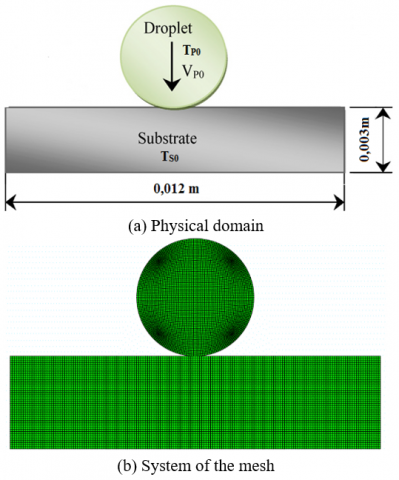Figure 1. Physical model under analysis

Table 1. Grid sensitivity study

 Time(s) 6000 nodes 11210 nodes 19660 nodes Coarse mesh Error (%) Medium mesh Error (%) Fine mesh Error (%) Experiment  2×10- 4 426 14.65 473 4.14 485 1.63 493 5×10- 4 437 14.11 488 3.02 502 0.20 503 8×10- 4 483 5.04 505 0.59 508 0.0 508 1×10- 3 515 0.97 513 0.58 512 0.39 510

The substrate and particle materials are considered to be isotropic elastic-plastic in this simulation, and as a result, the plastic deformation is estimated using Brief's formula.

$\sigma_{p l}=\sigma_y+\frac{E_X E_T}{E_X-E_T}\ \varepsilon_{P L}$     (11)

where, $E_x, E_T, \sigma_u, \varepsilon_{P L}$ stand for the Young modulus, tangent modulus, yield stress, and plastic strain, respectively.

The thermo-mechanical characteristics of the particle and substrate, which are temperature dependent, are taken into consideration in this numerical analysis and are available in References [29-33]. They can be summarized as follows in Tables 2 to 6. The yield strength and tangent modulus of the particle are not known at the melting point; therefore, they are taken to be one hundredth of the last values known.

It is common knowledge that there is no perfect contact between the splat and the substrate. The following expression is used to determine the thermal contact conductance (TCC) in the current simulation :

$T C C=1.45 \frac{k_s}{\sigma_r}\left(\frac{P}{H}\right)^{0.985}$     (12)

where, ks represents the thermal conductivity with a harmonic mean, the contact pressure is P, H is the softer material's microhardness and, the roughness of the surface is $\sigma_r$.

4. Results and Discussion

The current numerical model simulates the impact and deposition or flattening of a single aluminum particle on steel substrate during thermal spray process using a dynamic-temperature-displacement-explicit approach. We start off the first section of this study by validating the work that has already been done. The same issue was addressed using the volume of fluid method as well, Xue et al. . Figures 2 and 3 demonstrate that the behavior of the present results, particularly during the first moments of contact, behave similarly to those of Xue et al. . The two investigations used different approaches, which explains the discrepancy in the form and spread factor of the flattened particle.

At an initial temperature of 200℃, the results show the temperature progression of a 3.92 mm-diameter aluminum particle impacting on a tool steel substrate. Initial values of velocity and temperature of the particle are 3 m/s and 630℃, respectively. When the particle makes contact with the substrate, it spreads, solidifies, and takes on a lamellar shape.

Table 2. Mechanical properties of aluminum 6111

 Temperature (℃) 20 100 200 300 400 500 600 630 Young modulus (Gpa) 69.3 65.6 59.2 49.7 35.2 10.9 1 1 Poisson ratio 0.35 0.35 0.35 0.35 0.35 0.375 0.48 0.485 Yield stress (Mpa) 167 167 61.8 20.6 20.6 9.8 0.0098 0.00098 Tangent modulus (Mpa) 345 345 103 34.5 15 1.5 0.0015 0.0015

Table 3. Thermal properties of aluminum 6111

 Temperature (℃) 100 200 300 400 500 550 630 Thermal conductivity (w/m.℃) 144.5 147.5 152.5 148 135 128 110 Specific heat (j/kg.K) 978 1028 1078 1133 1159 1185 1211 Thermal expansion α10-5 (1/K) 2.28 2.35 2.47 2.57 2.69 2.75 2.89

Table 4. Latent heat, solidus and liquidus temperature of aluminum 6111

 Latent heat (j/Kg) Solidus temperature (℃) Liquidus temperature (℃) 389000 538 593

Density of AL 6111 is 2700 m3/kg.

Table 5. Mechanical properties of steel

 Temperature (℃) 21 93 204 316 427 538 649 Young modulus (Gpa) 207.6 207.6 194 186 169 117 55.1 Poisson ratio 0.28 0.28 0.28 0.28 0.28 0.28 0.28 Yield stress (Mpa) 248 238 224 200 172 145 75.8 Tangent modulus (Mpa) 2076 1964 1964 1860 1690 1690 75.8

Table 6. Thermal properties of steel 6111

 Temperature (℃) 21 93 204 316 427 538 649 Thermal conductivity (w/m℃) 64.8 63.31 55.38 49.99 44.9 39.81 34.95 Specific heat (j/kg.K) 450 450 500 500 500 500 520 Thermal expansion α10-5 (1/K) 1.1 1.15 1.22 1.3 1.35 1.4 1.46

According to the results, heat transfer from the particle to the substrate starts as soon as there is contact, and as a result, the temperature of the substrate beneath the splat surface increases from 200°C to 516°C in 0.6 ms before progressively decreasing; for more details, see Figure 4. Additionally, it is clear from Figure 4 that the maximum error between the experiment and simulation of Xue et al.  is about 15.62%. This difference is due to the assumption of using a constant melting point during simulation and the presence of contaminants and an oxide layer in experiment.

Figure 5 shows the contours of total displacement for various times. The particle starts to spread laterally as it makes contact with the substrate, creating a lamellar structure. It can be seen that the impact zone has a small displacement. The highest displacement always happens at the splat's edge. It is also evident that the particle's spreading begins to progressively slow down around about 2ms. The dissipation of the particle's kinetic energy through elastic and plastic deformation work results in a slowdown of the particle's velocity.

The Von-Mises stress over time is shown in Figure 6 to explain the mechanical interaction between the splat and the substrate. When a particle is in touch with the top face of the substrate, where the stress is initially stronger, the stress gradually spreads through the substrate over time.

Furthermore, it is clear from this figure that the substrate is subjected to higher stress than the particle. These findings can be explained by the different young modulus values for the two materials, as reported by Benramoul and El-Hadj .

A thin layer close to the surface experiences frictional shear as a result of particle spreading at the same level as the surrounding zone. There is residual tensile stress close to the surface as a result of the interplay of these layers. The XY shear stress contours are depicted in Figure 7. The findings demonstrate that shear stress grows over time with symmetry in intensity, with the maximum shear stress always occurring near the substrate's edge.

## figure_2a.png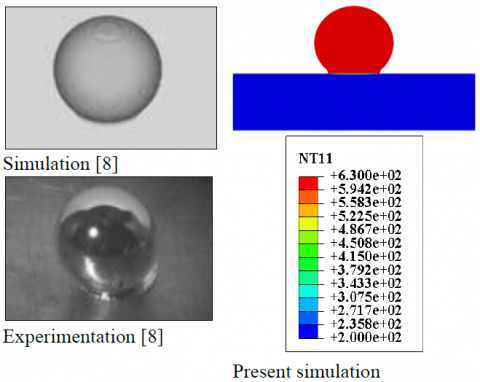(a) t=0.1 ms

## figure_2b.png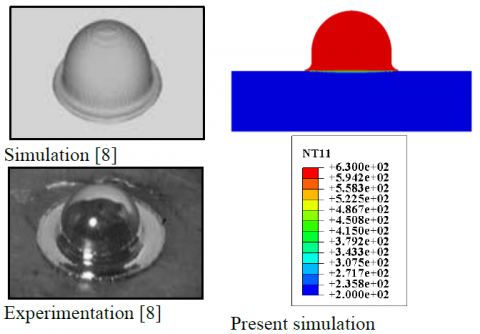(b) t=0.3 ms

## figure_2c.png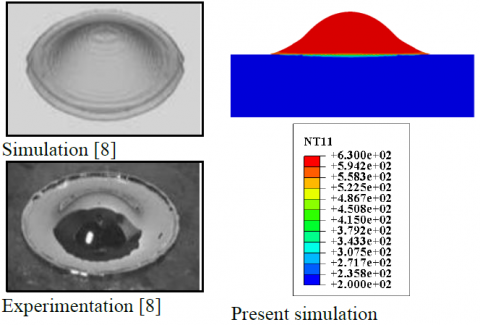(c) t=0.7 ms

## figure_2d.png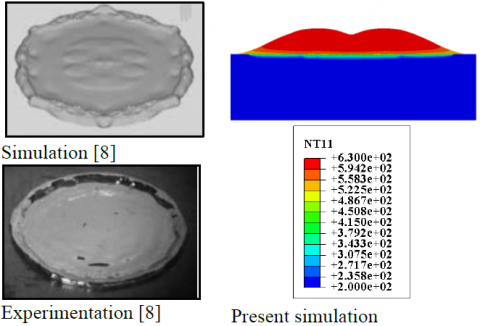(d) t=2.0 ms

## figure_2e.png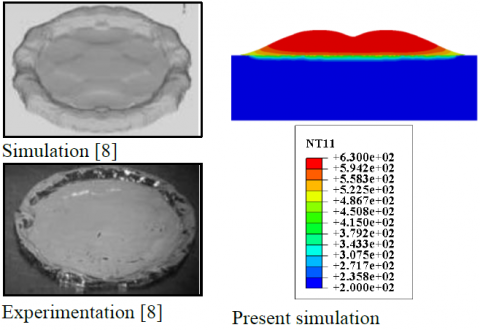(e) t=3.0 ms

## figure_2f.png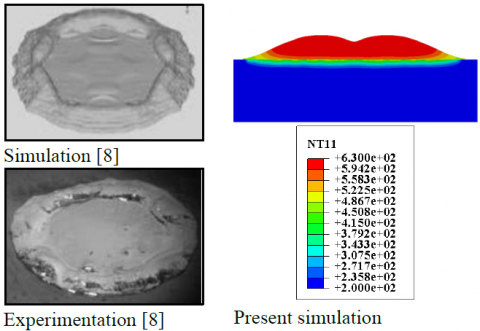(f) t=7.0 ms

Figure 2. Comparison of the numerical model used with other numerical and experimental models to validate the sequential effect of a 3.92mm aluminum drop

## figure_3.png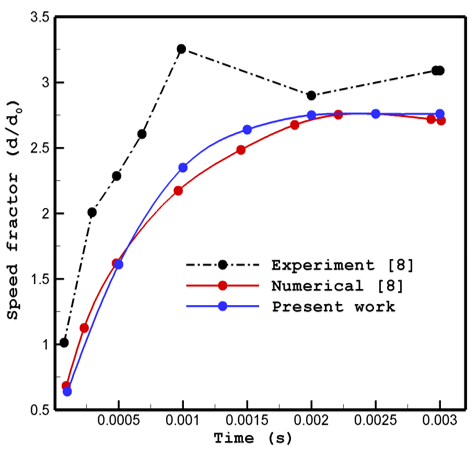Figure 3. Comparison of the spread factor between the current simulation's results and those reported in the literature for an aluminum droplet impacting at 3m/s

## figure_4.png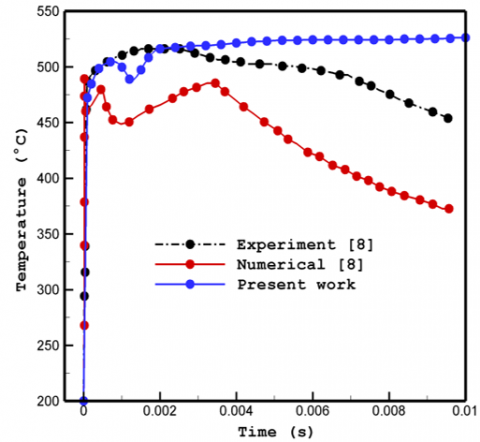Figure 4. Substrate surface temperature histories under the splat center

## figure_5a.png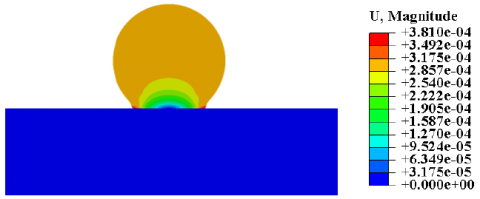(a) t=0.1 ms

## figure_5b.png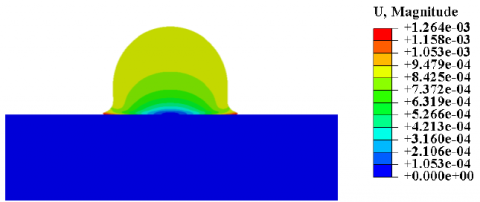(b) t=0.3 ms

## figure_5c.png(c) t=0.7 ms

## figure_5d.png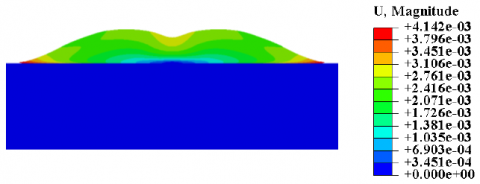(d) t=2.0 ms

## figure_5e.png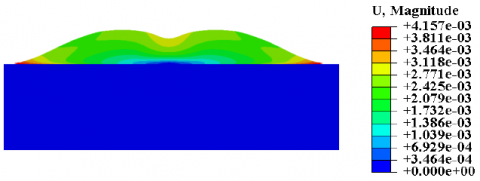(e) t=3.0 ms

## figure_5f.png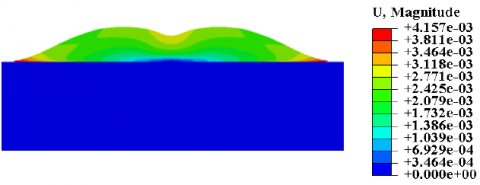(f) t=7.0 ms

Figure 5. Contour plots for displacement following successive impacts of a 3.92mm-diameter aluminum droplet impacting at a 3m/s impact velocity

## figure_6a.png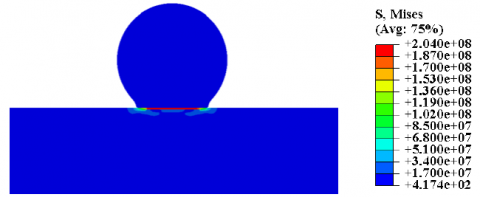(a) t=0.1 ms

## figure_6b.png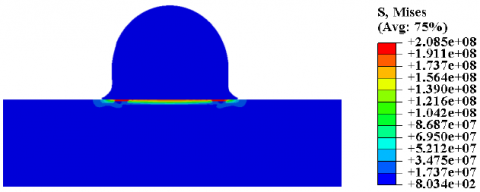(b) t=0.3 ms

## figure_6c.png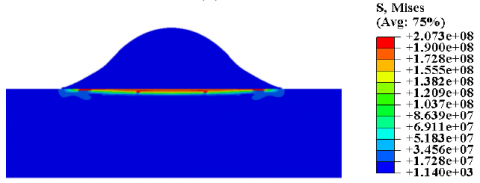(c) t=0.7 ms

## figure_6d.png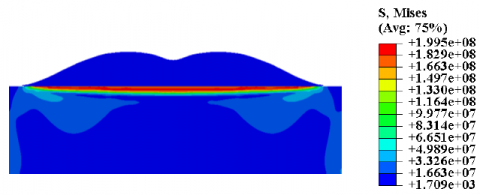(d) t=2.0 ms

## figure_6e.png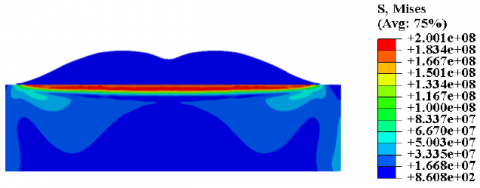(e) t=3.0 ms

## figure_6f.png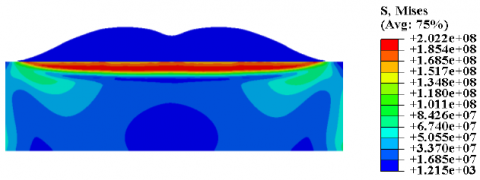(f) t=7.0 ms

Figure 6. Contour plots for Von mises stress following successive impacts of a 3.92mm-diameter aluminum droplet impacting at a 3m/s impact velocity

## figure_7a.png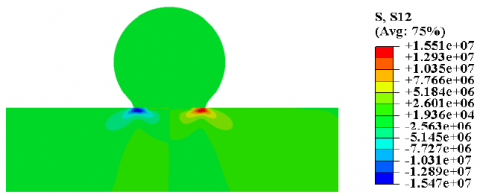(a) t=0.1 ms

## figure_7b.png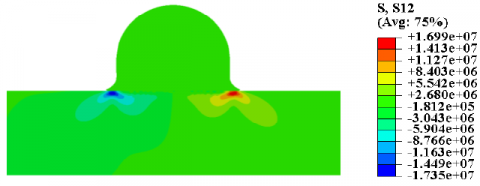(b) t=0.3 ms

## figure_7c.png(c) t=0.7 ms

## figure_7d.png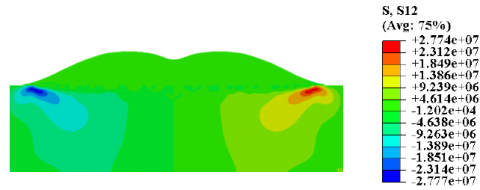(d) t=2.0 ms

## figure_7e.png(e) t=3.0 ms

## figure_7f.png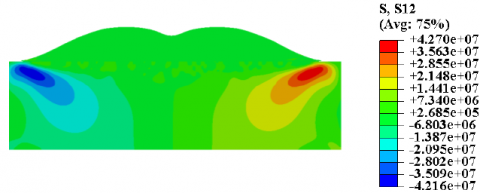(f) t=7.0 ms

Figure 7. Contour plots for XY shear stress following successive impacts of a 3.92mm-diameter aluminum droplet impacting at a 3m/s impact velocity

When a material is subjected to a load exceeding a threshold called the yield strength, it undergoes permanent and non-reversible deformation. The transition from elastic behavior to plastic behavior is known as yielding. Figure 8 displays the contours of the effective plastic strain (PEEQ). The plastic strain has larger values in the particle section than stress does. The impact zone of the particle is where the plasticity first manifests itself. Then, as the particle flattens, the plasticity region expands to encompass the entire particle. Additionally, it was discovered that the effective plastic strain had maximum values at both the center and the edges of the splat.

## figure_8a.png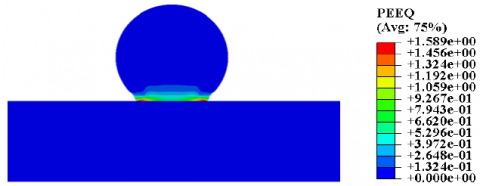(a) t=0.1 ms

## figure_8b.png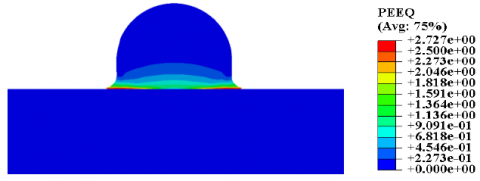(b) t=0.3 ms

## figure_8c.png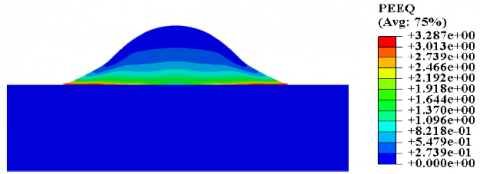(c) t=0.7 ms

## figure_8d.png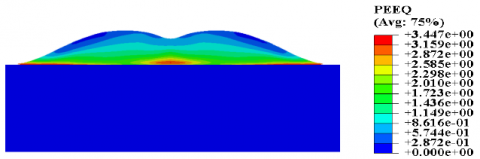(d) t=2.0 ms

## figure_8e.png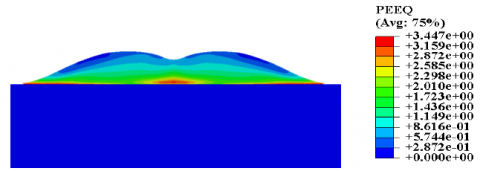(e) t=3.0 ms

## figure_8f.png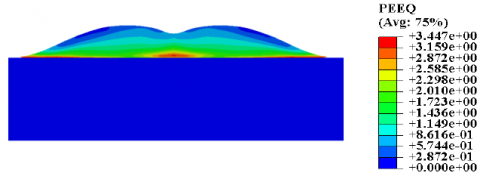(f) t=7.0 ms

Figure 8. Contour plots for PEEQ following successive impacts of a 3.92mm-diameter aluminum droplet impacting at a 3m/s impact velocity

Figure 9 showed the change in contact pressure over time at the particle's impact zone's center. In the first moments of contact, the contact pressure between the particle and the substrate rapidly increases and reaches a high of about 40 KPa. This low value is the result of the particle’s plastic deformation absorbing energy. Furthermore, contact pressure varies between positive and negative values, the opposite of the conclusions of the VOF model . The splat's adherence to the substrate is encouraged by the positive contact pressure. However, the negative value has a rebound impact on the particle, according to Benramoul and El-Hadj . Furthermore, the negative contact pressure at 0.002 s is most likely due to particle contraction during solidification or possibly the reaction of the substrate after getting impacted by the particle.

## figure_9.png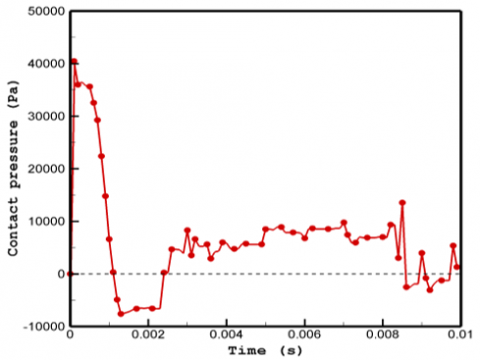Figure 9. The change in contact pressure at the particle's center contact point

5. Conclusions

This study simulates the impact and deposition of a single aluminum particle during the thermal spraying process. Instead of treating the particle as a fluid, a strategy based on a thermomechanical coupled model was employed. The model treats the particle as a solid with low or weak mechanical characteristics by reducing the yield stress and the particle's Young's modulus while increasing the Poisson's ratio. The set of governing equations is solved using the software Abaqus/explicit. The findings indicate that:

• Employing material property values that vary with temperature is suitable for modeling this type of problem.
• Incorporating a variable thermal contact conductance has a significant impact on the production of splats and heat transfer.
• The outcomes obtained from the present model agree well with the results obtained from the experiment and previous numerical data. As a result, the current model can be extended in future research to account for the effects of substrate movement and particle initial velocity on splat formation.
Nomenclature
 cp Specific heat (j/kg k) d0 Initial particle diameter (m) d Particle diameter (m) EX Young modulus (Pa) ET Tangent modulus (Pa) Hf Latent heat (j/Kg) H Micro hardness (Pa) Greek symbols σy Yield stress (Pa) εPL Plastic strain ρ Density (kg/m3) θN Temperature (℃) μN Degree of freedom β Degree of freedom σr Surface roughness (m)
References

 El-Hadj, A.A., Zirari, M., Bacha, N. (2010). Numerical analysis of the effect of the gas temperature on splat formation during thermal spray process. Applied Surface Science, 257(5): 1643-1648. http://dx.doi.org/10.1016/j.apsusc.2010.08.115

 Madejski, J. (1976). Solidification of droplets on a cold surface. International journal of heat and mass transfer, 19(9): 1009-1013. http://dx.doi.org/10.1016/0017-9310(76)90183-6

 Le Bot, C., Vincent, S., Meillot, E., Sarret, F., Caltagirone, J.P., Bianchi, L. (2015). Numerical simulation of several impacting ceramic droplets with liquid/solid phase change. Surface and Coatings Technology, 268: 272-277. http://dx.doi.org/10.1016/j.surfcoat.2014.10.047

 Shukla, R.K., Patel, V., Kumar, A. (2018). Modeling of rapid solidification with undercooling effect during droplet flattening on a substrate in coating formation. Journal of Thermal Spray Technology, 27(3): 269-287. http://dx.doi.org/10.1007/s11666-017-0666-y

 Xing, Y., Jiang, C., Hao, J., Sun, R. (2013). Numerical analysis on substrate melting during plasma-spraying cast iron on aluminum surface. Reviews on Advanced Materials Science, 33(3): 276-280.

 Haferl, S., Poulikakos, D. (2003). Experimental investigation of the transient impact fluid dynamics and solidification of a molten microdroplet pile-up. International Journal of Heat and Mass Transfer, 46(3): 535-550. http://dx.doi.org/10.1016/S0017-9310(02)00289-2

 Zhao, Y., Yu, Z., Planche, M.P., Lasalle, A., Allimant, A., Montavon, G., Liao, H. (2018). Influence of substrate properties on the formation of suspension plasma sprayed coatings. Journal of Thermal Spray Technology, 27(1): 73-83. http://dx.doi.org/10.1007/s11666-017-0671-1

 Xue, M., Heichal, Y., Chandra, S., Mostaghimi, J. (2007). Modeling the impact of a molten metal droplet on a solid surface using variable interfacial thermal contact resistance. Journal of Materials Science, 42(1): 9-18. https://doi.org/10.1007/s10853-006-1129-x

 Heichal, Y., Chandra, S. (2005). Predicting thermal contact resistance between molten metal droplets and a solid surface. Journal of Heat Transfer, 127(11): 1269-1275. http://dx.doi.org/10.1115/1.2039114

 Chung, M., Rangel, R.H. (2001). Parametric study of metal droplet deposition and solidification process including contact resistance and undercooling effects. International Journal of heat and mass Transfer, 44(3): 605-618. http://dx.doi.org/10.1016/S0017-9310(00)00112-5

 Zhang, Y., Matthews, S., Tran, A.T.T., Hyland, M. (2016). Effects of interfacial heat transfer, surface tension and contact angle on the formation of plasma-sprayed droplets through simulation study. Surface and Coatings Technology, 307: 807-816. http://dx.doi.org/10.1016/j.surfcoat.2016.09.066

 Zhang, H., Wang, X.Y., Zheng, L.L., Sampath, S. (2004). Numerical simulation of nucleation, solidification, and microstructure formation in thermal spraying. International Journal of Heat and Mass Transfer, 47(10-11): 2191-2203. http://dx.doi.org/10.1016/j.ijheatmasstransfer.2003.11.030

 Zirari, M., El-Hadj, A.A., Bacha, N. (2010). Numerical analysis of partially molten splat during thermal spray process using the finite element method. Applied Surface Science, 256(11): 3581-3585. ttp://dx.doi.org/10.1016/j.apsusc.2009.12.158

 Tong, A.Y., Holt, B.R. (1997). Numerical study on the solidification of liquid metal droplets impacting onto a substrate. Numerical Heat Transfer, Part A: Applications, 31(8): 797-817. http://dx.doi.org/10.1080/10407789708914065

 Ramanuj, V., Tong, A.Y. (2017). Simultaneous spreading and solidification of an impacting molten droplet with substrate remelting. Journal of Heat Transfer, 139(3): 032301. http://dx.doi.org/10.1115/1.4034813

 Wang, W., Hong, F.J., Qiu, H.H., Cheng, P. (2006). The impact of thermal contact conductance on the spreading and solidification of a droplet on a substrate. Heat Transfer Engineering, 27(9): 68-80. http://dx.doi.org/10.1080/01457630600846158

 Liao, Y., Zheng, Y., Zheng, Z., Li, Q. (2016). Numerical simulation of zirconia splat formation and cooling during plasma spray deposition. Applied Physics A, 122(7): 1-7. http://dx.doi.org/10.1007/s00339-016-0192-7

 Suli, L., Zhengying, W., Jun, D., Pei, W., Bingheng, L. (2017). A numerical analysis on the metal droplets impacting and spreading out on the substrate. Rare Metal Materials and Engineering, 46(4): 893-898. http://dx.doi.org/10.1016/S1875-5372(17)30118-2

 Zhang, Y., Matthews, S., Hyland M. (2017). Role of solidification in the formation of plasma sprayed nickel splats through simulation and experimental observation. International Journal of Heat and Mass Transfer, 115: 488-501. http://dx.doi.org/10.1016/j.ijheatmasstransfer.2017.07.072

 Benramoul, L., El-Hadj, A.A. (2011). An elastic-perfectly plastic model for simulating an aluminum particle behavior during plasma thermal spraying using the finite element method. Applied Surface Science, 258(2): 962-971. http://dx.doi.org/10.1016/j.apsusc.2011.09.039

 Danouni, S., El-hadj, A.A., Zirari, M., Belharizi, M. (2016). A thermo-mechanical analysis of a particle impact during thermal spraying. Applied Surface Science, 371: 213-223. http://dx.doi.org/10.1016/j.apsusc.2016.02.226

 Fardan, A., Ahmed, R. (2019). Modeling the evolution of residual stresses in thermally sprayed YSZ coating on stainless steel substrate. Journal of Thermal Spray Technology, 28(4): 717-736. http://dx.doi.org/10.1007/s11666-019-00856-2

 Xie, J., Nélias, D., Berre, W. L., Ogawa, K., Ichikawa, Y. (2015). Simulation of the cold spray particle deposition process. Journal of Tribology, 137(4): 41101-41115. https://doi.org/10.1115/1.4030257

 Koric, S., Thomas, B.G. (2006). Efficient thermo‐mechanical model for solidification processes. International Journal for Numerical Methods Inengineering, 66(12): 1955-1989. http://dx.doi.org/10.1002/nme.1614

 Yilbas, B.S., Akhtar, S., Shuja, S.Z. (2013). Laser forming and welding processes. Heidelberg: Springer International Publishing. http://dx.doi.org/10.1007/978-3-319-00981-0

 Chen, C., Kovacevic, R. (2004). Thermomechanical modelling and force analysis of friction stir welding by the finite element method. Proceedings of the Institution of Mechanical Engineers, Part C: Journal of Mechanical Engineering Science, 218(5): 509-519. https://doi.org/10.1243/095440604323052292

 Zhu, L., Jen, T.C., Pan, Y.T., Chen, H.S. (2017). Particle bonding mechanism in cold gas dynamic spray: A three-dimensional approach. Journal of Thermal Spray Technology, 26(8): 1859-1873. https://doi.org/10.1007/s11666-017-0652-4

 Özel, T., Zeren, E. (2005). Finite element method simulation of machining of AISI 1045 steel with a round edge cutting tool. In Proceedings of the 8th CIRP International Workshop on Modeling of Machining Operations, pp. 533-542.

 Kim, K.Y., Suzuki, T., Umeda, T. (1992). Deformation behaviour of al-si alloy castings and the associated metal mould. Cast Metals, 5(1): 42-50. http://dx.doi.org/10.1080/09534962.1992.11819091

 Miller, R.A. (2005). Prediction of part distortion in die casting (No. Final Technical Report). The Ohio State University (US).

 Wang, J., Wang, H.P., Lu, F., Carlson, B.E., Sigler, D.R. (2015). Analysis of Al-steel resistance spot welding process by developing a fully coupled multi-physics simulation model. International Journal of Heat and Mass Transfer, 89: 1061-1072. http://dx.doi.org/10.1016/j.ijheatmasstransfer.2015.05.086

 Canas, J., Picon, R., Pariis, F., Blazquez, A., Marin, J.C. (1996). A simplified numerical analysis of residual stresses in aluminum welded plates. Computers & Structures, 58(1): 59-69. https://doi.org/10.1016/0045-7949(95)00112-T

  Zhang, C., Tang, L. (2017). Measuring elasto-plastic properties of solid wall materials of metallic foams at elevated temperatures by using indentation hardness technique. Materials at High Temperatures, 34(1): 41-44. http://dx.doi.org/10.1080/09603409.2016.1232682

 Cooper, M.G., Mikic, B.B., Yovanovich, M.M. (1969). Thermal contact conductance. International Journal of Heat and Mass Transfer, 12(3): 279-300. http://dx.doi.org/10.1016/0017-9310(69)90011-8